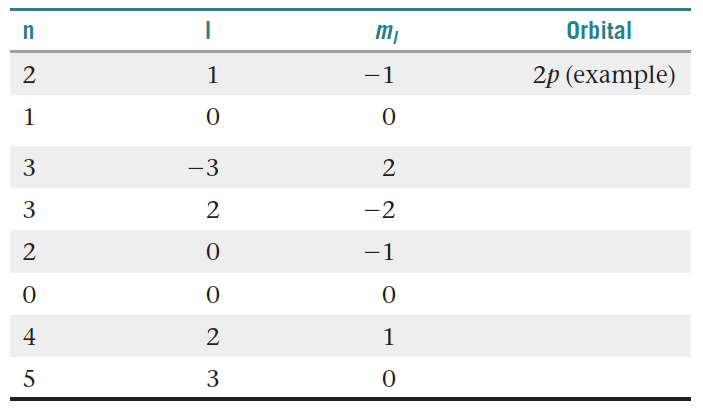# Problem: You may want to reference(Pages 227 - 231)Section 6.5 while completing this problem.For the table that follows, indicate which orbital corresponds to each set of quantum numbers. Ignore x, y, and z subscripts. If the quantum numbers are not allowed (i.e., contain an illegitimate value), indicate that by using the "not allowed" label.

🤓 Based on our data, we think this question is relevant for Professor Cole & Geng & Lovander's class at IOWA.

###### FREE Expert Solution

We are asked to determine the orbitals which correspond to the values of n, l, and ml values given.

 n l ml Orbital 2 1 -1 2p (example) 1 0 0 3 3 2 3 2 -2 2 0 -1 0 0 0 4 2 1 5 3 0

Let’s first define the values of first three quantum numbers:

principal quantum number (n)  energy level in orbitals and its value could be any positive integer starting from 1

angular momentum quantum number (ℓ)  (l) has to be at least 1 less than n, range of values from 0 up to (n-1) and each number corresponds to a subshell:###### Problem Details

You may want to reference(Pages 227 - 231)Section 6.5 while completing this problem.

For the table that follows, indicate which orbital corresponds to each set of quantum numbers. Ignore x, y, and z subscripts. If the quantum numbers are not allowed (i.e., contain an illegitimate value), indicate that by using the "not allowed" label.